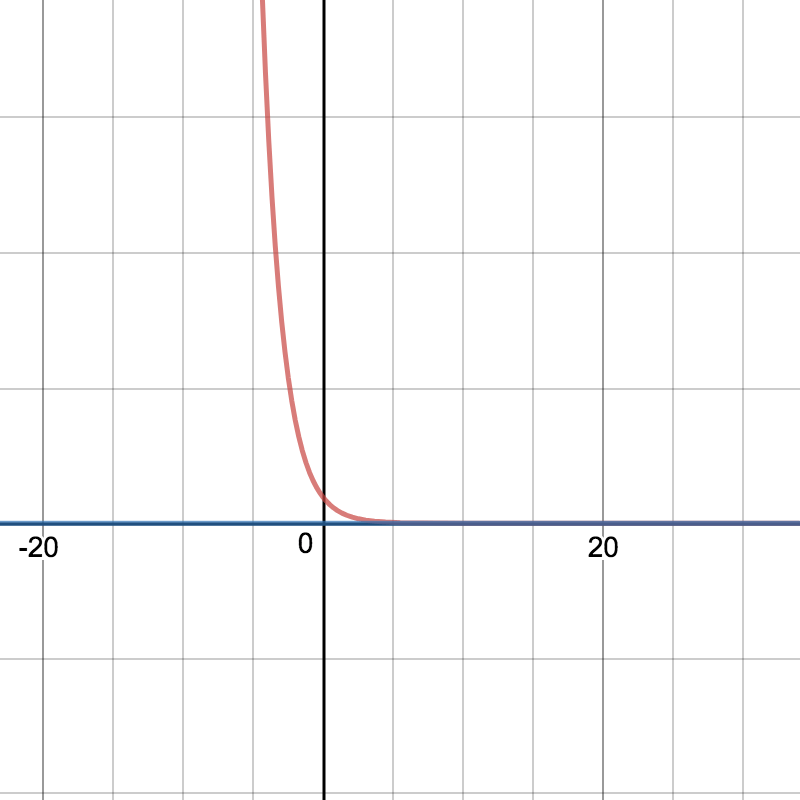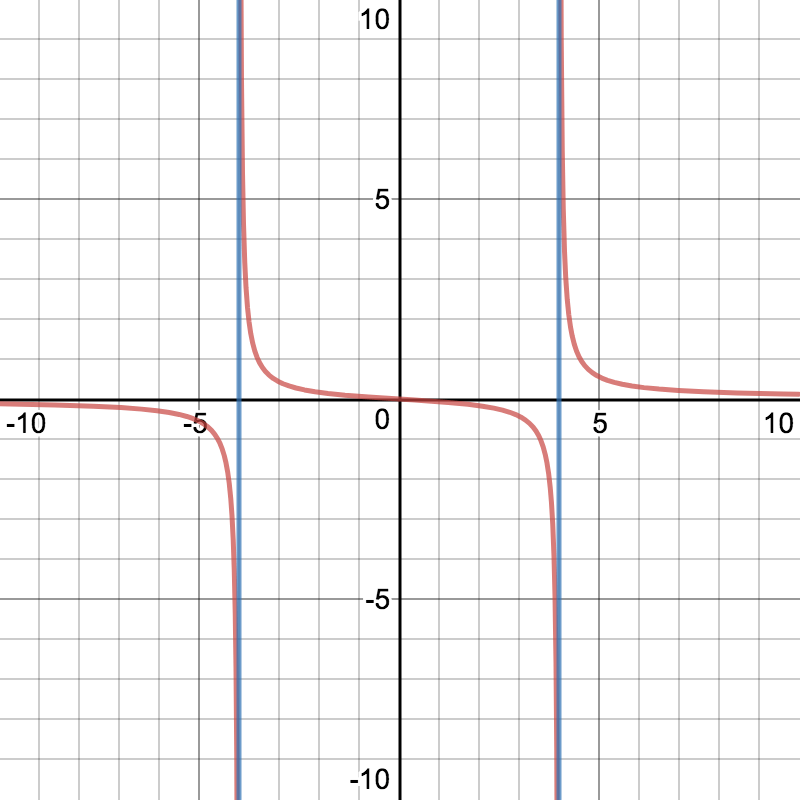# RATL 3 | Lesson 3 | Explore (Asymptotes)# Asymptotes

Knowing the zeros gives us some information about the shape of the graph. It is also helpful to know the location of the asymptotes, if there are any.  What is an asymptote?  An asymptote is a line that a curve approaches, but never reaches.Note that the function gets close to, but does not cross, the line $$y = 0$$Note that in this graph the function gets close to $$x=-4$$ and $$x=4$$ but does not cross either of these lines.

To determine if a rational function has vertical or horizontal asymptotes you will need to know the following:

Horizontal Asymptotes (HA):

1. If the degree of the polynomial is greater in the numerator than in the denominator, the rational function has no horizontal asymptote.
2. If the degree of the polynomial is greater in the denominator than in the numerator, the rational function has a horizontal asymptote at $$y=0$$.
3. If the degree of the polynomial in the numerator is the same as the degree of the polynomial in the denominator, then the horizontal asymptote is the ratio of the leading coefficients.

Vertical Asymptotes (VA):

Vertical asymptotes are found by setting the denominator equal to zero. Any rational roots are vertical asymptotes. If the denominator has no real roots, then the rational function has no zeros.